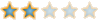# Week 2 Problem D##Week 2 Problem D

Note from Archis: This problem is separated into three sections. I have bolded the questions, and for the first section you are allowed to use Google to find the boiling points and freezing points. If you have memorized them that is accepted too.

While pleasant spring days, sunshine and rising temperatures are helping to warm you up physically, this week's problems will help warm you up mathematically. First, trivia questions: What is the freezing temperature for water in Celsius and Fahrenheit? What is the boiling temperature for water in Celsius and Fahrenheit?

Who Came Up With Those Nasty Formulas, Anyway? Have trouble remembering which temperature conversion formula contains a 9/5 (or a 5/9), which one adds 32 (and which one subtracts it), and which one needs parentheses (and which one doesn't)? For reference, the formulas are F = 9/5 C + 32 and C = 5/9 (F - 32) Oish. No easy task remembering those. But we're here to help. Here are two formulas for converting temps that look enough alike that they're easy to remember. They are: C = 5/9 (F + 40) - 40 and F = 9/5 (C + 40) - 40 Both use parentheses. Both have a +40 and a -40. You just need to remember which one gets which fraction, and that shouldn't be too hard. Use these "new" formulas to convert 40 degrees Fahrenheit to Celsius and to convert 50 degrees Celsius to Fahrenheit.

Greater Equality: For what numerical value is the Celsius temperature equal to the Fahrenheit temperature?

##Re: Week 2 Problem D

That's a cool problem. I'll post my solution laterSteve
The Cool CubePosts : 85
Points : 4
Reputation : 4
Join date : 2008-10-07
Age : 22
Location : ...herePermissions in this forum:
You cannot reply to topics in this forum